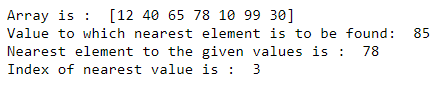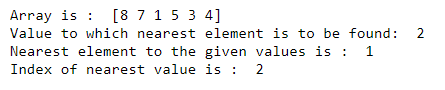GeeksforGeeks App
Open AppBrowser
Continue

# Find the nearest value and the index of NumPy Array

In this article, let’s discuss finding the nearest value and the index in an array with Numpy. We will make use of two of the functions provided by the NumPy library to calculate the nearest value and the index in the array. Those two functions are numpy.abs() and numpy.argmin()

Example

```Input Array: [12 40 65 78 10 99 30]
Nearest value is to be found: 85

Nearest values: 78
Index of nearest value: 3```

### Approach to Find the nearest value and the index of NumPy Array

• Take an array, say, arr[] and an element, say x to which we have to find the nearest value.
• Call the numpy.abs(d) function, with d as the difference between the elements of array and x, and store the values in a different array, say difference_array[].
• The element, providing minimum difference will be the nearest to the specified value.
• Use numpy.argmin(), to obtain the index of the smallest element in difference_array[]. In the case of multiple minimum values, the first occurrence will be returned.
• Print the nearest element, and its index from the given array.

### Example 1:

To find the nearest element to the specified value 85. We subtract the given value from each element of the array and store the absolute value in a different array. The minimum absolute difference will correspond to the nearest value to the given number. Thus, the index of minimum absolute difference is 3 and the element from the original array at index 3 is 78.

## Python3

 `import` `numpy as np` `# array``arr ``=` `np.array([``12``, ``40``, ``65``, ``78``, ``10``, ``99``, ``30``])``print``(``"Array is : "``, arr)` `# element to which nearest value is to be found``x ``=` `85``print``(``"Value to which nearest element is to be found: "``, x)` `# calculate the difference array``difference_array ``=` `np.absolute(arr``-``x)` `# find the index of minimum element from the array``index ``=` `difference_array.argmin()``print``(``"Nearest element to the given values is : "``, arr[index])``print``(``"Index of nearest value is : "``, index)`

Output:### Example 2:

In this example, The minimum absolute difference will correspond to the nearest value to the given number. Thus, the index of minimum absolute difference is 2 and the element from the original array at index 2 is 1. Thus, 1 is nearest to the given number i.e 2.

## Python3

 `import` `numpy as np` `# array``arr ``=` `np.array([``8``, ``7``, ``1``, ``5``, ``3``, ``4``])``print``(``"Array is : "``, arr)` `# element to which nearest value is to be found``x ``=` `2``print``(``"Value to which nearest element is to be found: "``, x)` `# calculate the difference array``difference_array ``=` `np.absolute(arr``-``x)` `# find the index of minimum element from the array``index ``=` `difference_array.argmin()``print``(``"Nearest element to the given values is : "``, arr[index])``print``(``"Index of nearest value is : "``, index)`

Output:My Personal Notes arrow_drop_up